## #StackBounty: #r #regression #logistic #bayesian log-odds and it's standard error as priors in logistic regression

### Bounty: 50

I’m attempting to complete a Bayesian logistic regression with the outcome of whether or not a crash occurred. I have various covariates in my model that are widely used to predict crash occurrence. As such, I’m using informed priors from prior publications that report the odds ratio and it’s 95% C.I for each covariate.

Here’s an example of a prior provided by the model I’m pulling from

crash at night (OR 13.1; 95% CI 5.0 to 31.5) : log-odds (1.12,.20) from $$frac{log(31.5-5)}{3.92}$$

I wanted to apply the log-odds of these results and their standard error in my updated model as priors. My first thought was to apply the log-odds and it’s a standard error on a normal prior. I’m using logic from the sources 1 & 2 listed at the end of the post.

My question, if my assumptions about applying these log-odds and SE’s on a normal prior are correct, can I simply transform the SE of the log odds to variance and implement?

a normal prior:

βk = (μβk2βk)

requires a variance rather than an SE. According to citation 3 log-odds SE and be transformed into log-odds VAR:

$$SE[log(OR)] = sqrt{VAR[log(OR)]} => SE^2 = VAR[log(OR)]$$

therefore, if I square the standard error x then I should be able to apply this as my final prior:

βk = (1.12,.04)

Is this assumption correct or am I way off? Is there a better way of implementing log-odd priors and their SE’s into a logistic regression model?

Thanks!

1. AdamO (https://stats.stackexchange.com/users/8013/adamo), Prior for Bayesian multiple logistic regression, URL (version: 2016-03-16): https://stats.stackexchange.com/q/202046

"Basically, you have the flexibility to parametrize estimation however
you see fit, but using a model which is linear on the log odds scale
makes sense for many reasons. Furthermore, using a normal prior for
log odds ratios should give you very approximately normal posteriors."

1. Sander Greenland, Bayesian perspectives for epidemiological research: I. Foundations and basic methods, International Journal of Epidemiology, Volume 35, Issue 3, June 2006, Pages 765–775, https://doi.org/10.1093/ije/dyi312

"To start, suppose we model these a priori ideas by placing 2:1 odds
on a relative risk (RR) between ½ and 2, and 95% probability on RR
between ¼ and 4. These bets would follow from a normal prior for the
log relative risk ln (RR) that satisfies…"

1. StatsStudent (https://stats.stackexchange.com/users/7962/statsstudent), How do I calculate the standard deviation of the log-odds?, URL (version: 2020-04-19): https://stats.stackexchange.com/q/266116

Get this bounty!!!

## #StackBounty: #r #regression #logistic #bayesian log-odds and it's standard error as priors in logistic regression

### Bounty: 50

I’m attempting to complete a Bayesian logistic regression with the outcome of whether or not a crash occurred. I have various covariates in my model that are widely used to predict crash occurrence. As such, I’m using informed priors from prior publications that report the odds ratio and it’s 95% C.I for each covariate.

Here’s an example of a prior provided by the model I’m pulling from

crash at night (OR 13.1; 95% CI 5.0 to 31.5) : log-odds (1.12,.20) from $$frac{log(31.5-5)}{3.92}$$

I wanted to apply the log-odds of these results and their standard error in my updated model as priors. My first thought was to apply the log-odds and it’s a standard error on a normal prior. I’m using logic from the sources 1 & 2 listed at the end of the post.

My question, if my assumptions about applying these log-odds and SE’s on a normal prior are correct, can I simply transform the SE of the log odds to variance and implement?

a normal prior:

βk = (μβk2βk)

requires a variance rather than an SE. According to citation 3 log-odds SE and be transformed into log-odds VAR:

$$SE[log(OR)] = sqrt{VAR[log(OR)]} => SE^2 = VAR[log(OR)]$$

therefore, if I square the standard error x then I should be able to apply this as my final prior:

βk = (1.12,.04)

Is this assumption correct or am I way off? Is there a better way of implementing log-odd priors and their SE’s into a logistic regression model?

Thanks!

1. AdamO (https://stats.stackexchange.com/users/8013/adamo), Prior for Bayesian multiple logistic regression, URL (version: 2016-03-16): https://stats.stackexchange.com/q/202046

"Basically, you have the flexibility to parametrize estimation however
you see fit, but using a model which is linear on the log odds scale
makes sense for many reasons. Furthermore, using a normal prior for
log odds ratios should give you very approximately normal posteriors."

1. Sander Greenland, Bayesian perspectives for epidemiological research: I. Foundations and basic methods, International Journal of Epidemiology, Volume 35, Issue 3, June 2006, Pages 765–775, https://doi.org/10.1093/ije/dyi312

"To start, suppose we model these a priori ideas by placing 2:1 odds
on a relative risk (RR) between ½ and 2, and 95% probability on RR
between ¼ and 4. These bets would follow from a normal prior for the
log relative risk ln (RR) that satisfies…"

1. StatsStudent (https://stats.stackexchange.com/users/7962/statsstudent), How do I calculate the standard deviation of the log-odds?, URL (version: 2020-04-19): https://stats.stackexchange.com/q/266116

Get this bounty!!!

## #StackBounty: #probability #bayesian #mathematical-statistics #posterior #conjugate-prior Help with the prior distribution

### Bounty: 50

The question is as follows:

Consider an SDOF mass-spring system. The value of the mass is known and is equal to 1 kg.
The value of the spring stiffness is unknow and based on the experience and judgement the following is assumed. Value of stiffness is in the following range [0.5, 1.5] N/m.

To have a more accurate estimate of the value of the stiffness an experiment is performed where in the natural frequency of the system is observed. The following observation are made:

``````  Observation 1     Freq = 1.021 rad/sec
Observation 2     Freq = 1.015 rad/sec
Observation 3     Freq = 0.994 rad/sec
Observation 4     Freq = 1.005 rad/sec
Observation 5     Freq = 0.989 rad/sec
``````
1. Based on the information provided write the functional form of prior PDF.
2. Plot the likelihood function with different number of observations.
3. Based on the information provided write the functional form of the posterior PDF.
4. Plot the posterior distribution.

My work so far:

spring constant $$k = sqrt{{w}/{m}}$$
m = 1kg, so $$w = k^{2}$$.

$$k sim Uniform(0.5, 1.5)$$,

so pdf of w = $$f(w) = 2w$$

where $$w epsilon [sqrt{0.5},sqrt{1.5}]$$

So prior distribution is linear in the range root(0.5), root(1.5).

$$Likelihood = L = 2^{5}(1.0211.015..0.989) approx 2.04772$$

This is what I have done so far. I am new to Bayesian inference and I am not sure how to proceed after this or if what I have done so far is correct. Pleas advice on how to find the posterior function.

Get this bounty!!!

## #StackBounty: #bayesian #bootstrap #posterior How well does weighted likelihood bootstrap approximate the Bayesian posterior?

### Bounty: 50

$$DeclareMathOperator*{argmax}{arg,max}$$Given a set of $$N$$ i.i.d. observations $$X=left{x_1, ldots, x_Nright}$$, we train a model $$p(x|boldsymbol{theta})$$ by maximizing marginal log-likelihood $$log p(X mid boldsymbol{theta})$$. A full posterior $$p(boldsymbol{theta}|X)$$ over model parameters $$boldsymbol{theta}$$ can be approximated as a Gaussian distribution using Laplace method.

In the case that the Gaussian distribution gives a poor approximation of $$p(boldsymbol{theta}|X)$$, Newton and Raftery (1994) proposed weighted likelihood bootstrap (WLB) as a way to simulate approximately from a posterior distribution. Extending Bayesian bootstrap (BB) of Rubin (1981), this method generates BB samples $$tilde{X}=(X,boldsymbol{pi})$$ by repeatedly drawing sampling weights $$boldsymbol{pi}$$ from a uniform Dirichlet distribution and maximizes a weighted likelihood to calculate $$boldsymbol{theta}_{text{MWLE}}$$.

$$begin{equation} boldsymbol{theta}{text{MWLE}}=argmax{boldsymbol{theta}}sum_{n=1}^{N} pi_nlog p(x_n|boldsymbol{theta}). end{equation}$$

So the algorithm can be summarized as

• Draw a posterior sample $$boldsymbol{pi}sim p(boldsymbol{pi}|X)=mathcal{D}ir(1,dots,1)$$.
• Calculate $$theta_{text{MWLE}}$$ from weighted sample $$tilde{X}=(X, boldsymbol{pi})$$

Newton and Raftery (1994) state that

In the generic weighting scheme, the WLB is first order correct under
quite general conditions.

1. I was wondering what exactly does this mean and what does first order refer to? How well does this approximation $$p(boldsymbol{theta}|X)$$?

Later authors state that

Inaccuracies can be removed by using the WLB as a source of samples in
the sampling-importance resampling (SIR) algorithm.

1. I was not sure what this exactly means. Could someone point what step in my algorithm exactly should I change?

Get this bounty!!!

## #StackBounty: #machine-learning #classification #probability #bayesian Problem understanding probabilistic generative models for classi…

### Bounty: 50

I am a student and I am studying machine learning. I am focusing on probabilistic generative models for classification and I am having some troubles understanding this topic.

In the slide of my professor it is written the following: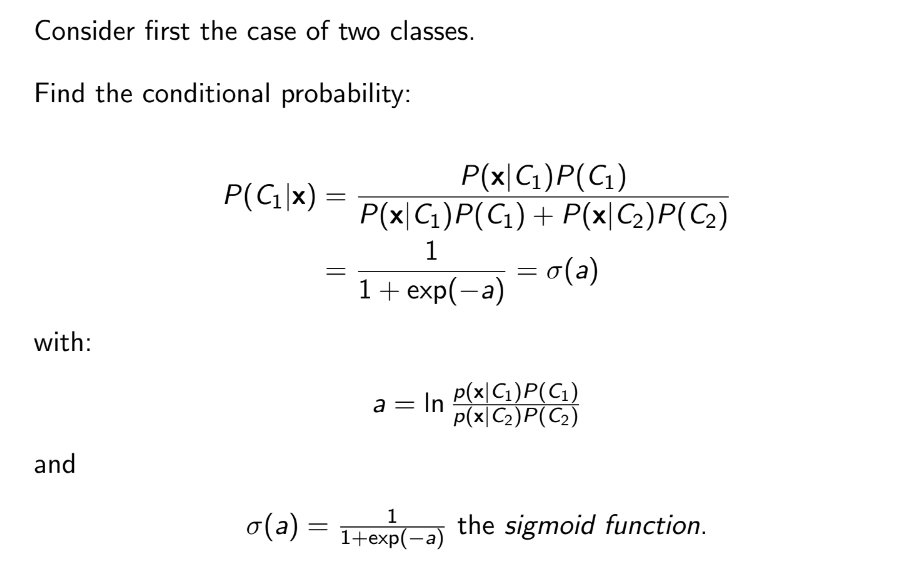which I don’t understand.

So far, I have understood that in the generative probailistic models, we ant to estimate $$P(C_i|x)$$, which is the probability of having class $$i$$ given a data $$x$$, using the likelihood and the Bayes theorem.

So, it starts by writing the Bayes rule, but the the slides says that we can write this as a sigmoid, but why?

If I have to try to give an answer to it, I would say because the sigmoid gives a number from $$0$$ to $$1$$, and so a probability, but it is just a guess I am doing.

Moreover, it continues by saying that we can use a gaussian distribution for $$P(x|C_i)$$, and so $$P(x|C_i)=N(mu ,sigma )$$, and so :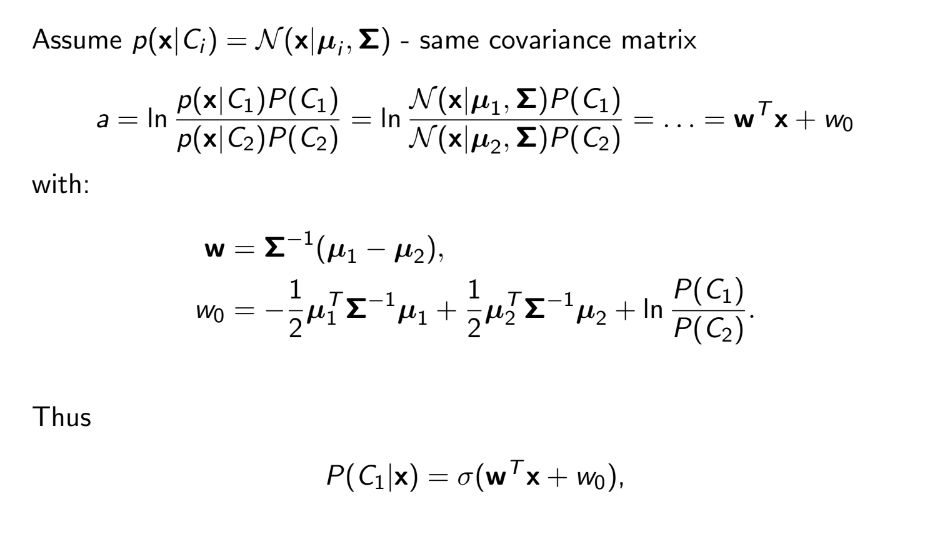I don’t know if my question is clear so sorry if it is not but I am really confused. If it is not lcear please tell me I will try to edit it. Thanks in advance.

Note: if it can be useful, this has been taken from the Bishop book at page 197

Get this bounty!!!

## #StackBounty: #bayesian #mcmc #differential-equations #hmc How does Hamiltonian Monte Carlo work?

### Bounty: 50

I made the below graphic to explain how I currently understand the HMC algorithm. I’d like verification from a subject matter expert if this understanding is or isn’t correct. The text in the below slide is copied below for ease of access:

Hamiltonian Monte Carlo: A satellite orbits a planet. The closer the satellite is to the planet, the greater the effects of gravity. This means, (A) higher potential energy and (B) higher kinetic energy needed to sustain orbit. That same kinetic energy at a further distance from the planet, would eject the satellite out from orbit. The satellite is tasked with collecting photos of a specific geographic region. The closer the satellite orbits the planet, the faster it moves in orbit, the more times it passes over the region, the more photographs it collects. Conversely, the further a satellite is from the planet, the slower it moves in orbit, the less times it passes over the region, the less photographs it collects.
In the context of sampling, distance from the planet represents distance from the expectation of the distribution. An area of low likelihood is far from the expectation; when “orbiting this likelihood,” lower kinetic energy means less samples collected over a fixed interval of time, whereas when orbiting a higher likelihood means more samples collected given the same fixed time interval.
In a given orbit, the total energy, kinetic and potential, is constant; however, the relationship between the two is not simple. Hamiltonian equations relate changes in one to the other. Namely, the gradient of position with respect to time equals momentum. And the gradient of momentum with respect to time equals the gradient of potential energy with respect to position. To compute how far a satellite will have traveled along its orbital path, leapfrog integration must be used, iteratively updating momentum and position vectors. In the context of sampling, likelihood is analogous to distance from the planet and the gradient of potential energy with respect to position is the gradient of the probability density function with respect to its input parameter, x. This information allows the orbital path around various inputs, X, corresponding to the same likelihood, y, to be explored.
However, we’re not simply interested in exploring one likelihood, we must explore multiple orbital paths. To accomplish this, the momentum must randomly be augmented, bringing the satellite closer or further away from the planet. These random “momentum kicks” allow for different likelihoods to be orbited. Fortunately, hamiltonian equations ensure that no matter the likelihood, the number of samples collected is proportionate to the likelihood, thus samples collected follow the shape of the target distribution.

My question is – Is this an accurate way to think about how Hamiltonian Monte Carlo works?Edit:

I’ve implemented in some code based on my understanding of the algorithm. It works for a gaussian with mu=0, sigma=1. But if I change sigma it breaks. Any insights would be appreciated.

``````import numpy as np
import random
import scipy.stats as st
import matplotlib.pyplot as plt

def normal(x,mu,sigma):
numerator = np.exp((-(x-mu)**2)/(2*sigma**2))
denominator = sigma * np.sqrt(2*np.pi)
return numerator/denominator

def neg_log_prob(x,mu,sigma):
num = np.exp(-1*((x-mu)**2)/2*sigma**2)
den = sigma*np.sqrt(np.pi*2)
return -1*np.log(num/den)

def HMC(mu=0.0,sigma=1.0,path_len=1,step_size=0.25,initial_position=0.0,epochs=1_000):
# setup
steps = int(path_len/step_size) -1 # path_len and step_size are tricky parameters to tune...
samples = [initial_position]
momentum_dist = st.norm(0, 1)
# generate samples
for e in range(epochs):
q0 = np.copy(samples[-1])
q1 = np.copy(q0)
p0 = momentum_dist.rvs()
p1 = np.copy(p0)
dVdQ = -1*(q0-mu)/(sigma**2) # gradient of PDF wrt position (q0) aka momentum wrt position

# leapfrog integration begin
for s in range(steps):
p1 += step_size*dVdQ/2 # as potential energy increases, kinetic energy decreases
q1 += step_size*p1 # position increases as function of momentum
p1 += step_size*dVdQ/2 # second half "leapfrog" update to momentum
# leapfrog integration end
p1 = -1*p1 #flip momentum for reversibility

#metropolis acceptance
q0_nlp = neg_log_prob(x=q0,mu=mu,sigma=sigma)
q1_nlp = neg_log_prob(x=q1,mu=mu,sigma=sigma)

p0_nlp = neg_log_prob(x=p0,mu=0,sigma=1)
p1_nlp = neg_log_prob(x=p1,mu=0,sigma=1)

# Account for negatives AND log(probabiltiies)...
target = q0_nlp - q1_nlp # P(q1)/P(q0)
adjustment = p1_nlp - p0_nlp # P(p1)/P(p0)

event = np.log(random.uniform(0,1))
if event <= acceptance:
samples.append(q1)
else:
samples.append(q0)

return samples
``````

Now it works here:

``````mu, sigma = 0,1
trial = HMC(mu=mu,sigma=sigma,path_len=2,step_size=0.25)

# What the dist should looks like
lines = np.linspace(-6,6,10_000)
normal_curve = [normal(x=l,mu=mu,sigma=sigma) for l in lines]

# Visualize
plt.plot(lines,normal_curve)
plt.hist(trial,density=True,bins=20)
plt.show()
``````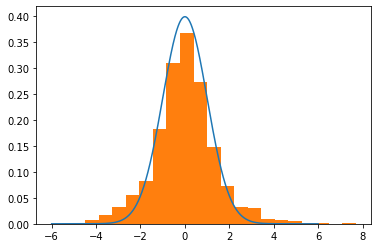But it breaks when I change sigma to 2.

``````# Generate samples
mu, sigma = 0,2
trial = HMC(mu=mu,sigma=sigma,path_len=2,step_size=0.25)

# What the dist should looks like
lines = np.linspace(-6,6,10_000)
normal_curve = [normal(x=l,mu=mu,sigma=sigma) for l in lines]

# Visualize
plt.plot(lines,normal_curve)
plt.hist(trial,density=True,bins=20)
plt.show()
``````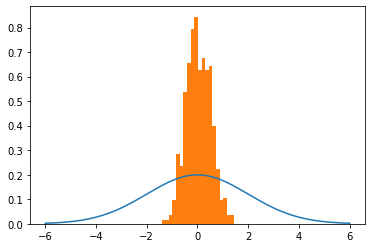Any ideas? I feel like I’m close to "getting it".

Get this bounty!!!

## #StackBounty: #bayesian #bootstrap Understanding Bayesian Bootstrap theory

### Bounty: 50

I’m trying to understand the theory in section 4 of Rubin (1981) paper on Bayesian Bootstrap (BB):

$$textbf{Theory:}$$ Let $$d=left(d_{1}, ldots, d_{K}right)$$ be the vector of all possible distinct values of $$X$$, and let $$pi=left(pi_{1}, cdots, pi_{K}right)$$ be the associated vector of probabilities
$$Pleft(X=d_{k} mid piright)=pi_{k}, quad sum pi_{k}=1$$
Let $$x_{1}, ldots, x_{n}$$ be an i.i.d. sample from the equation above and let $$n_{k}$$ be the number of $$x_{i}$$ equal to $$d_{k}$$. If the prior distribution of $$pi$$ is proportional to
$$prod_{k=1}^{K}pi_{k}^{l_k}quad left(0right. text { if } left.sumpi_{k} neq 1right)$$
then the posterior distribution of $$pi$$ is the $$K-1$$ variate Dirichlet distribution $$Dleft(n_{1}+l_{1}+1,right.$$ $$left.ldots, n_{K}+l_{K}+1right)$$ which is proportional to
$$quad prod_{k=1}^{K} pi_{k}^{left(n_{k}+l_{k}right)} quadleft(0right. text{ if } x_{imath} neq d_{k} text{for some } i, k text{ or if} left.sum pi_{k} neq 1right)$$

• What does $$K-1$$ variate mean?

This posterior distribution can be simulated using $$m-1$$ independent uniform random numbers, where $$m=n+K+sum_{1}^{K} l_{k}$$.

• Where does this come from?

Let $$u_{1}, cdots, u_{m-1}$$ be i.i.d. $$U(0,1),$$ and let $$g_{1}, cdots, g_{m}$$ be the $$m$$ gaps generated by the ordered $$u_{imath}$$. Partition the $$g_{1}, cdots, g_{m}$$ into $$K$$ collections, the $$k$$-th having $$n_{k}+l_{k}+1$$ elements,

• Is element referring to $$u$$‘s or gaps? I think gaps because $$sum_1^K(n_{k}+l_{k}+1)=m$$. If so, is partitioning mean to group adjacent gaps together? Something like the bottom line below for $$m=7$$ and $$K=3$$?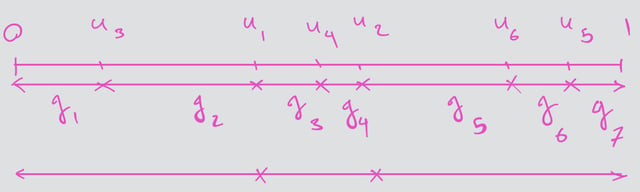and let $$P_{k}$$ be the sum of the $$g_{i}$$ in the $$k$$-th collection, $$k=1, cdots, K$$.

• Does this mean $$P_{k}$$ is the size of collection $$k$$? Does "sum of the $$g_{i}$$" mean sum of the length of $$g_{i}$$‘s?

Then $$left(P_{1}, ldots, P_{K}right)$$ follows the $$K-1$$ variate $$Dleft(n_{1}+l_{1}+1, ldots, n_{K}+l_{K}+1right)$$ distribution. Consequently, the BB which assigns one gap to each $$x_{i}$$

• But we have $$m$$ gaps vs. $$n$$ $$x_i$$‘s. How does this work?

is simulating

• What does simulating mean in this context?

the posterior distribution of $$pi$$ and thus of a parameter $$phi=Phi(pi, d)$$ under the improper prior distribution proportional to $$prod_{k=1}^{K} pi_{k}^{-1}$$.

• Where did the $$l_k=-1$$ come from?

Simulations corresponding to other prior distributions with integer $$l_{k}$$ can also be performed; for example, with a uniform prior distribution on $$pi$$, (i.e., all $$l_{k}=0$$ ) generate $$n+K-1$$ uniform random variables, form $$n+K$$ gaps, add the first $$left(n_{1}+1right)$$ gaps together to yield the simulated value of $$pi_{1}$$, add the second $$left(n_{2}+1right)$$ gaps together to yield the simulated value of $$pi_{2}$$, and so on. However, when using a proper prior distribution, all a priori possible values of $$X$$ must be specified because they have positive posterior probability.

• What does "all a priori possible values of $$X$$ must be specified" mean and how is this different from the previous case of improper prior with $$l_k=-1$$?

Get this bounty!!!

## #StackBounty: #bayesian #normal-distribution #variance #gamma-distribution #inverse-gamma Bayesian estimation of the variance

### Bounty: 50

The mean of the Gamma distribution is $$alpha/beta$$, while the mean of the Inverse Gamma is $$beta/(alpha-1)$$. Similarly, the mode of the Gamma is $$(alpha-1)/beta$$, but the mode of the Inverse Gamma is $$alpha/(beta+1)$$.

How does this relate to the title of the question?

Well, if we are given data $$X$$ assumed to be normally distributed, the population variance is given by:
$$sigma_{pop}^2=frac{sum(x_i-bar x)^2}{n}equivfrac{s_n^2}{n}$$
However, if we take a Bayesian approach and choose a non-informative Normal-Inverse-Gamma conjugate prior for the variance (i.e. $$alpha_0rightarrow0, beta_0rightarrow0$$), we have that the marginal distribution of $$sigma^2$$ is also Inverse-Gamma distributed, with $$alpha=n/2, beta=s_n^2/2$$ and mean:
$$E[sigma^2] = frac{s_n^2/2}{n/2-1}neqsigma_{pop}^2 quad(!)$$
On the other hand, if one uses an uninformative Normal-Gamma prior:
$$E[tau] = frac{n/2}{s_n^2/2} = frac{1}{sigma_{pop}^2}$$

Assuming the above is correct, I have a couple of questions:

1. I realize that $$E[1/X]neq1/E[X]$$, yet I’m not sure why $$E[tau]$$ specifically should yield the "correct" result. What’s wrong with using $$E[sigma^2]$$?
2. The frequentist approach would lead to using the sample variance, which seems to match neither approaches with an uninformative prior. What if any, is the significance of the particular prior that would result in the sample variance for both $$tau$$ and $$sigma$$?
3. What is the significance of the mode, i.e. the MAP estimator of $$sigma^2$$ or $$tau$$? again, they are both different, and I don’t believe I’ve seen either being used in practice.

Get this bounty!!!

## #StackBounty: #regression #bayesian #multiple-regression #censoring #rstan How best to deal with a left-censored predictor (because of …

### Bounty: 200

Context: I’m new to Bayesian stats and am trying to fit a multiple regression with rstan. All variables are continuous and there is no hierarchical structure.

One of my predictors is left-censored because it falls below the detection limit for a chemical assay. What is the best way to deal with this in a multiple regression? So far, I can see a few possibilities:

1. A substitution rule, such as ‘replace all values below the detection limit with a constant such as detection limit/2’. This is clearly not rigorous.
2. Multiple imputation, but (i) I don’t know how to deal with the fact that values above the detection limit are likely to be generated by the imputation process, which I will know with high probability to be false, and (ii) I’m not sure how well multiple imputation plays with Bayesian approaches, since I can’t think of a good way to aggregate the posterior distributions from fits to the different imputed datasets
3. Simulate values data from a distribution that makes sense based on prior knowledge and the data, and randomly assign values below the detection limit to the relevant points. This suffers from similar problems to #2, since I would have to simulate many sets of values, model them separately, and then figure out how to integrate the posteriors.

Am I missing better options? Are there useful Bayesian tricks that can help deal with this problem? I’m also open to non-Bayesian options.

The histogram below shows the distribution of values. The plot is on a log scale because that is most natural for this variable. For visual clarity, I have treated values below the detection limit (~25% of the data) as being 1/10 of the detection limit, and added a red line to separate them from the remaining points. Note that the red line is not the precise detection limit; the smallest quantified values to the right of the red line are at the putative limit. The fact that there are very few values exactly at the limit suggests that there may have been some variation in the detection limit between measurements, but I don’t mind if that is ignored for the purposes of this question.Get this bounty!!!

## #StackBounty: #self-study #bayesian #continuous-data #uniform #analytical Two dependent uniformly distributed continuous variables and …

### Bounty: 50

I am trying to solve the following exercise from Judea Pearl’s Probabilistic Reasoning in Intelligent Systems: Networks of Plausible Inference.

2.2. A billiard table has unit length, measured from left to right. A ball is rolled on this table, and when it stops, a partition is placed at its stopping position, a distance $$x$$ from the left end of the table. A second ball is now rolled between the left end of the table and the partition, and its stopping position, $$y$$, is measured.

a. Answer qualitatively: How does knowledge of $$y$$ affect our belief about $$x$$? Is $$x$$ more likely to be near $$y$$ , far from $$y$$, or near the midpoint between $$y$$ and 1?

b. Justify your answer for (a) by quantitative analysis. Assume the stopping position is uniformly distributed over the feasible range.

For b., I clearly need to use Bayes’ theorem:

$$P(X|Y) = dfrac{P(Y|X)P(X)}{P(Y)}$$

where I expressed

$$P(X) sim U[0,1] = begin{cases} 1, text{where } 0 leq x leq 1\ 0, text{else} end{cases} \ P(Y|X) sim U[0,x] = begin{cases} 1/x, text{where } 0 leq y leq x\ 0, text{else} end{cases}$$

I tried getting $$P(Y)$$ by integrating the numerator over $$X$$.

$$int_{-infty}^{infty} P(Y|X)P(X)dx = int_{0}^{1}P(Y|X)cdot 1 dx = int_{0}^{1}dfrac{1}{x} dx$$

But the integral doesn’t converge.

I also tried to figure out the numerator itself, but I don’t see how $$frac{1}{x}$$ can represent $$P(X|Y)$$.

Where did I go wrong?

Get this bounty!!!